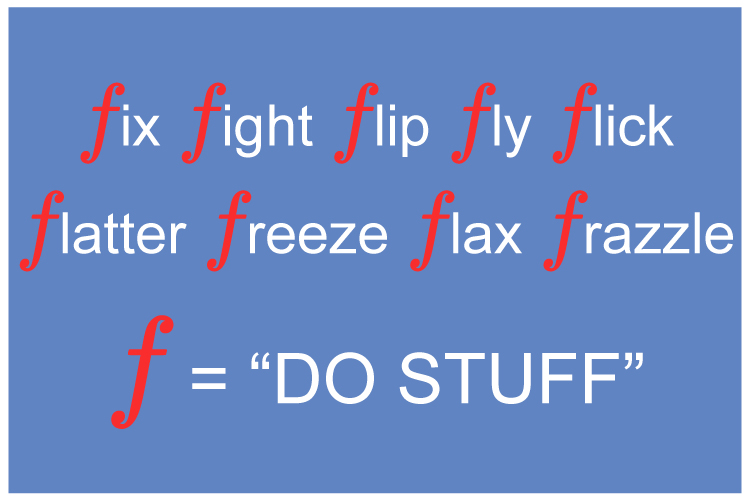# Function of X

f(x) means function of x.

Which means "Do stuff" to x.NOTE:

What stuff you do and what x  is, massively changes what happens.

f(x)                     means do stuff to x.

f(x)=x+4             means the stuff you do to x  is add 4.

f(2)                     means do stuff to x when x=2.

NOTE:

In maths thef can be a g  or an h  or any letter. (Really? Yes really)

Example 1

Given f(x)=3x+2  find f(2)

This is solved as follows:

f(x)=3x+2  means the "Stuff" you do to x is multiply it by 3  and then add 2.

f(2)  means "Do stuff" to x  when x=2.

So   f(x)=3x+2

f(2)=3(2)+2

f(2)=6+2

f(2)=8

Example 2

Given f(x)=x+4  find f(3)

This is solved as follows:

f(x)=x+4  means the "Stuff" you do to x is add 4.

f(3)  means "Do stuff" to x  when x=3.

So   f(x)=x+4

f(3)=3+4

f(3)=7

Example 3

Given f(x)=3x-1  find f(-2)

This is solved as follows:

f(x)=3x-1  means the "Stuff" you do to x is multiply it by 3  and then subtract 1.

f(-2)  means "Do stuff" to x  when x=-2.

So   f(x)=3x-1

f(-2)=3(-2)-1

f(-2)=-6-1

f(-2)=-7

NOTE:

This type of question can be phrased by giving the answer first, see below:

Example 4

If f(x)=15-4x  calculate the value of x  when f(x)=35.

This just says that

15-4x=35

Therefore

-4x=35-15

-4x=20

x=20/-4=-5